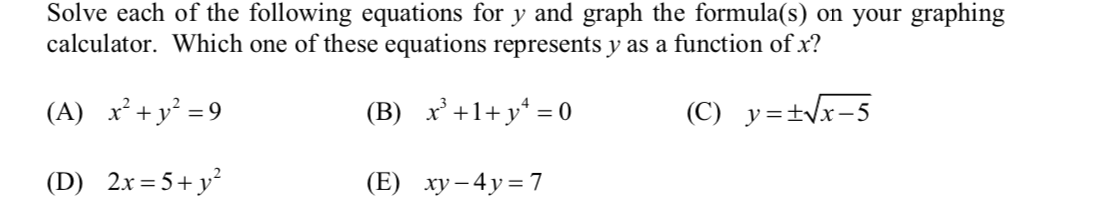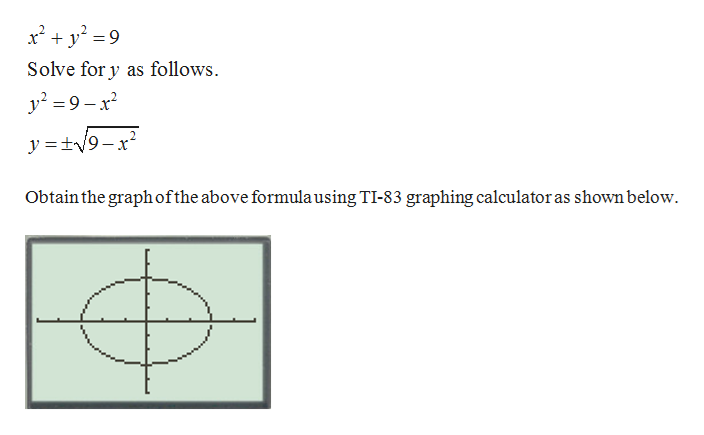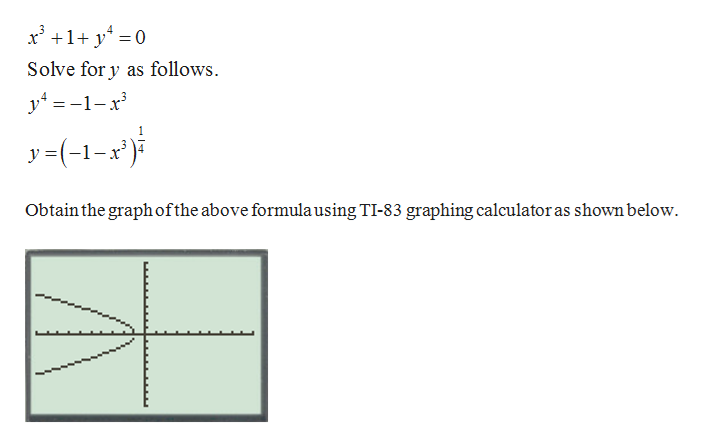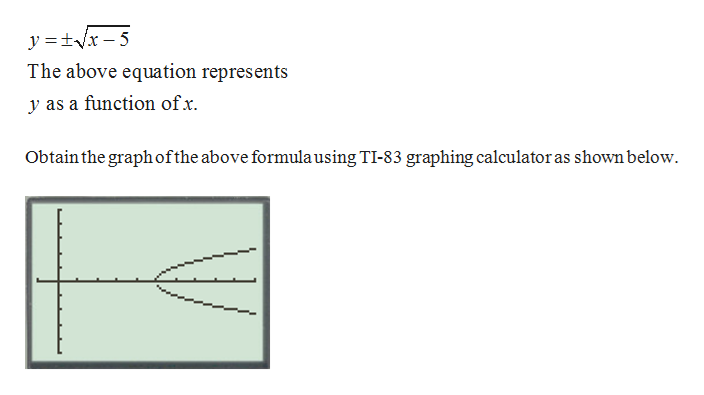# Solve each of the following equations for y and graph the formula(s) on your graphingcalculator. Which one of these equations represents y as a function of x?(A) x²+y² = 9x’+1+ y* = 0(C) y=±Vx-5(B)2.x = 5+ y?(E) ху -4у%3D7(D)

Question
4 viewshelp_outlineImage TranscriptioncloseSolve each of the following equations for y and graph the formula(s) on your graphing calculator. Which one of these equations represents y as a function of x? (A) x²+y² = 9 x’+1+ y* = 0 (C) y=±Vx-5 (B) 2.x = 5+ y? (E) ху -4у%3D7 (D) fullscreen
check_circle

Step 1

(A) The given function is,help_outlineImage Transcriptionclosex² + y° =9 Solve for y as follows. y? = 9 – x y =±V9-x? Obtain the graphofthe above formulausing TI-83 graphing calculator as shown below. fullscreen
Step 2

(B) The given function is,help_outlineImage Transcriptionclosex' +1+ y* =0 Solve for y as follows. y4 = -1-x %3D y=(-1-x') Obtain the graphofthe above formula using TI-83 graphing calculator as shown below. fullscreen
Step 3

(C) The given fun...help_outlineImage Transcriptionclosey =+Vx – 5 The above equation represents y as a function of x. Obtain the graphofthe above formulausing TI-83 graphing calculator as shown below. fullscreen

### Want to see the full answer?

See Solution

#### Want to see this answer and more?

Solutions are written by subject experts who are available 24/7. Questions are typically answered within 1 hour.*

See Solution
*Response times may vary by subject and question.
Tagged in

### Other